### Complex Power and Power Triangle

Introduction

In the last article we discussed the basics of Electrical Power and related terminologies. Here we will develop the concept of  Complex Power and Power Triangle. These two are very important concepts used frequently by power engineers. As this article requires the knowledge of previous article so it is advised to at least have a look  at the last article.

From the previous article the following points are clear.
• The instantaneous power p is composed of real power and reactive power.
• The time average value of instantaneous power is the real power (true power) is |V | | I | cos φ
• The instantaneous reactive power oscillates about the horizontal axis, so its average value is zero
• The maximum value of the reactive power is |V | | I | sin φ

It should be remembered that real power is the average value and the reactive power is maximum value.

Complex Power

In power system analysis the concept of Complex Power is frequently used to calculate the real and reactive power.

This is a very simple and important representation of real and reactive power when voltage and current phasors are known. Complex Power is defined as the product of Voltage phasor and conjugate of current phasor. See Fig-A

Let voltage across a load is represented by phasor V  and  current through the load is I.

If S is the complex power then,

S = V . I*

V is the phasor representation of voltage and I* is the conjugate of current phasor.

So if  V is the reference phasor then V can be written as |V| ∠0.
(Usually one phasor is taken reference which makes zero degrees with real axis. It eliminates the necessity of introducing a non zero phase angle for voltage)

Let current lags voltage by an angle φ, so  I = | I | ∠-φ
(current phasor makes -φ degrees with real axis)

I*=  | I | ∠φ
So,
S = |V|  | I | ∠(0+φ) =  |V|  | I | ∠φ

(For multiplication of phasors we have considered polar form to facilitate calculation)

Writting the above formula for S in rectangular form we get

S =  |V|  | I | cos φ  +  j  |V|  | I | sin φ

The real part of complex power S is |V| | I | cos φ which is the real power or average power and the imaginary part  |V| | I | sin φ is the reactive power.

So,              S = P + j Q

Where        P = |V| | I | cos φ    and    Q = |V| | I | sin φ

It should be noted that S is considered here as a complex number. The real part P is average power which is the average value, where as imaginary part is reactive power which is a maximum value. So I do not want to discuss further and call S as phasor. If you like more trouble I also advise you to read my article about phasor  or some other articles on phasor and complex numbers.

Returning to the main point, from the above formula it is sure that P is always more than zero. Q is positive when φ is positive or current lags voltage by φ degrees. This is the case of inductive load. We previously said that inductance and capacitance do not consume power. The power system engineers often say about reactive power consumption and generation. It is said that inductive loads consume reactive power and capacitors produce reactive power. This incorrect terminology creates confusion.

The fact is that most of the loads are inductive and they unnecessarily draw more current from source. Although in each cycle both inductance and capacitance draw power from the source and return same amount of power to the source but the behavior of inductance and capacitance are opposing to each other. When capacitors are connected in parallel to inductive load the power requirement of inductive load is supplied by capacitor in half cycle and in next half cycle the reverse happens. Depending upon the values of capacitor this power requirement of inductance in the load may be fully or partially satisfied. If partially satisfied the rest will be drawn from the distant source. By properly selecting the capacitance the maximum value of reactive power (Q) drawn from the distant source (or returned to the distant source) is reduced. This reduction in reactive power results in reduction of line current so the reduction of losses in transmission line and improvement in voltage at load end.

Power Triangle

Returning to the complex power formula, P, Q and S are represented in a power triangle as shown in figure below.

S is the hypotenuse of the triangle, known as Apparent Power. The value of apparent power is |V|| I |

or    |S| = |V|| I |

It is measured in VoltAmp or VA.

P is measured in watt and Q is measured in VoltAmp-Reactive or VAR. In power systems instead of these smaller units larger units like Megawatt, MVAR and MVA is used.

The ratio of real power and apparent power is the power factor of the load.

power factor = Cos φ = |P| / |S|

= |P| / √(P 2+Q 2)

The reactive power Q and apparent power S are also important in power system analysis. As just shown above the control of reactive power is important to maintain the voltage within the allowed limits. Apparent power is important for rating the electrical equipment or machines.

Total Power of Parallel Circuits

In real world the loads are usually connected in parallel. Here we will show the total power consumed by parallel branches. See Figure-C. It has two branches.

First we have to draw the individual power triangles for each branch. Next the power triangles are arranged back to back keeping real power in positive x direction as shown. The total power consumed is obtained by connecting starting point O to the tip of last triangle. This is actually the result of addition of complex numbers.

If   S= P+j Q1
S= P+j Q2

Then,  S = S1+  S

or    S = (P1+  P ) + j  (Q1+  Q)

P = P1+  P
Q = Q1+  Q

In the above diagram S1  P1 ,   Q1 and φ 1 correspond to branch1 and S P2 ,   Q2 and φ  correspond to branch2.
S, P, Q and φ correspond to total power consumption as seen by the generator

### Instantaneous, Average, Real and Reactive Power

Forward

We discussed and developed some important concepts of transmission lines in last few articles. Last time we discussed about long transmission lines. Here we discuss a simple but important basic concept Electric Power. This will refresh our knowledge before we move further.

Electric Power has same meaning as mechanical power but here the power or energy that we are concerned is in Electrical form. We often encounter terms like instantaneous, average, total, real, reactive, apparent and complex power or simply power. What they mean? how are they related ? That we will discuss here and in next article.

DC Circuit

As long as our analysis is restricted to Direct Current(DC) circuit the power consumed by the resistance load is the product of voltage across the resistance and current flowing through the resistance. It is really simple.

P = V . I
The power consumed by the load is the product of voltage across the load and current drawn by the load (Fig-A). Or the Power supplied by the DC source (battery/cell) is the product of voltage across the cell and current supplied by the cell. Both are equal in our example figure(considering ideal battery of zero internal resistance). The law of energy conservation implies power supplied by the source must be same as power consumed by the circuit. In DC circuit case instantaneous power is same as average power.
AC Circuit

In AC circuit analysis, what is this power that we talk about. The main problem is that the AC voltage and current varies sinusoidally with time. Moreover the presence of circuit reactive elements like Inductor and capacitor shift the current wave with respect to voltage wave (angle of phase difference).

Power is rate at which energy is consumed by load or produced by generator. Whether it is DC circuit or AC circuit, the value of instantaneous power is obtained by multiplying instantaneous voltage with instantaneous current. If at any instant of time t the voltage and current values are represented by sine functions as

v = Vm  sin ωt

i = Im  sin (ωt-φ)

Vm and Im  are the maximum values of the sinusoidal voltage and current. Here ω=2 π f
f is the frequency and ω is the angular frequency of rotating voltage or current phasors. It should be clear that for a power system f is usually 50 or 60 Hz
φ is the phase difference between the voltage and current.

As we said the instantaneous power is the product of instantaneous voltage and current, if we name instantaneous power as p then

p = v.i =  Vm  sin ωt  .  Im  sin (ωt-φ)
or  p = Vm Im  sin ωt  sin (ωt-φ)

Applying trigonometric formula 2.sin A.sin B = cos(A-B) - cos (A+B)  we get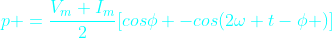It can be written as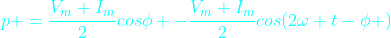This is the equation of instantaneous power

In the Fig-C is drawn all the three waves corresponding to v, i and p. Graphically also we can get the value of instantaneous power (p) at any instant of time t by simply multiplying the value of current i and voltage v at that particular instant t. (You can verify that in the diagram p is negative when either v or i is negative otherwise p is positive. See the points where p is zero). In the graph we have shown horizontal axis as angle φ instead of time t for easy visualization. It should be clear that both way it is correct.

Clearly the instantaneous power p is composed of two terms. The first term is constant because for a given load the phase angle φ is fixed. It does not change unless the load is changed.  The second term  is varying with time sinusoidally due to the presence of the term cos (2ωt-φ). Look that the instantaneous power frequency is twice the frequency of voltage or current.

So the instantaneous power in a single phase circuit varies sinusoidally.

The instantaneous power,  p = constant term + sinusoidal oscillating term.

In one complete period the average of oscillating term is zero.

Then what is the average power within a given time, say one Time Period of the wave?

It is the constant term.

Here is another way to think about the average power.
Just observe that the instantaneous power is negative for a small time. For any time interval you just find the total +ve area A+ (above horizontal-axis (blue line) and below p curve) and total -ve area A- (below horizontal axis and  above p curve). The net area is obtained by subtracting A- from A+. By dividing this net area ( by the time interval T we get the average power(P). You can do this using calculus. What you will ultimately get is only the first term in the above formula for instantaneous power p.

In still another way it is easier to realize that the formula for instantaneous power p has a constant term  (Vm.Im / 2) cos φ and the other sinusoidal term (Vm.Im / 2) cos (2 wt - φ). Actually p is the oscillating power which oscillates about the average constant term  (Vm.Im / 2) cos φ .

So the average power is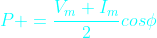The above formula can be written as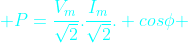Or,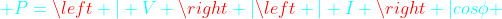here,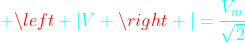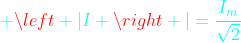V and I are the phasor representation of RMS values* of voltage and current sinusoids. The symbols |V| and |I| are  the magnitudes of phasors V and I. (See at the buttom for definition of RMS value).

This above formula is your favorite formula for useful power that we are most concerned about. This average power formula is used to find the power consumed by the load. The monthly electric energy bill at home is based on this power. The engineers and technicians in power or electrical industry simply use the term power instead of average power. So whenever we simply call power it means average power.

Of course the instantaneous power is oscillating in nature. As we already said it does not oscillates about the horizontal-axis rather about the average power P (cyan color horizontal line).

P will be zero when cos φ =0 or  φ  = 90 degree, that is when the phase angle between voltage and current waves is 90 degrees. It is only when the load is pure inductive or capacitive. In this case the second term only remains in the instantaneous power formula.

From the above figure for some time the power becomes negative that means the load supply energy to source for this period. This is due to the presence of reactive element in load.

The above formula for instantaneous power can be written in another form. This form actually is an attempt to distinguish the oscillating reactive power from the instantaneous power formula.   Rearranging the terms in equation for instantaneous power above we get

p = |V| | I | cos φ (1-cow2ωt) - |V| | I | sin φ sin2ωt

In this equation the first term |V| | I | cos φ (1-cow2ωt) is oscillatory whose average value is |V| | I | cos φ. We already talked about this average power.

The second term |V| | I | sin φ sin2ωt which is also oscillatory but with zero average value. The maximum value of this term is |V| | I | sin φ. This is the so called Reactive power. So Reactive power is the maximum value of a oscillatory power that is repeatedly drawn from the source and again returned to the source within each cycle. So the average of this reactive power is zero.

The average power P is called as Real Power. It is also sometimes called active power.

Real power = P = |V| | I | cos φ

It is usually written as P = VI cos φ. But it should be remembered that V and I are the rms values of voltage and current. For example when we say single phase 220 volt AC it means the rms value of voltage is 220 volts ( it is not maximum value of voltage sinusoid)

Reactive power = Q = |V| | I | sin φ

Real power is measured in Watt and the reactive power is measured in VAR (VoltAmpereReactive). In power sector these units are too small so real power is measured in Megawatt (MW) and reactive power in Megavar (MVAR). The letter R at the end denotes reactive power.

Many times students and practicing engineers are confused about the average power (often simply called power). They think that what they get by multiplying RMS voltage and RMS current is RMS power. No that is wrong. There is no RMS power. RMS power has no meaning or not defined. (Also see definition of RMS value, below at the end). It is average power or real power or true power.

Power In Three phase Balanced System

Let us consider a three phase balanced system. A three phase balanced system is analysed considering only one phase and neutral return. This is called per phase analysis. So the above analysis for single phase is true for balanced three phase case. Let the total power here is Pt. Then we get total three phase power as thrice of single phase case.

P= 3 |V| | I | cos φ

It should be remembered that |V| and | I |  are the per phase values. and φ is the phase angle of load in per phase analysis.

The above formula for balanced three phase system can be written as

P= √3 |Vl| | Il | cos φ

In the above formula Vl and  Il are line voltage and current (Fig-D). This equation is independent of type of three phase load connection i.e delta or star connected load. You have to know the line voltage, line current and phase angle φ as above. This form is very convenient and used often in power calculation.

There is one main difference between the single phase and total three phase power. The instantaneous single phase power is pulsating. In the balanced three phase case, each phase instantaneous power is pulsating but the three pulsating power waves are 120 degrees displaced from each other. At any instant of time the total of these three instantaneous power waves is a constant which is 3 |V| | I | cos φ. So the total power consumed in three phase balanced system is not pulsating. Non-pulsating power also imply the desired non-pulsating torque in case of three phase rotating machines. In large 3-phase motors this is really desired.

*RMS value of AC Sinusoids

The value of AC voltage or current that produces the same heating (or same energy) that is produced if DC voltage or current numerically equal to RMS value of AC is applied instead of AC. This concept helps make the formula for power similar for both DC and AC circuits.

You should read the next article about Power Triangle and Complex Power